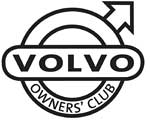# Volvo 440 460 480 VIN Plates

1800  |  200  |  300  |  400  |  700  |  850 - 900  |  40/50  |  60  |  70  |  80  |  90  |  XC90

This information will help you to understand the information on the VIN plate of your Volvo car.

Click on the model series number above

Example VIN Number:

XLDEX041HCG123456

XLD E X 4 1 H C G 123456
Manufacturer Code Vehicle Type Safety Equipment Engine Type Gearbox Fixed Digit Model Year Factory Code Chassis Number

Manufacturer Code
YV1 = cars YV2 = trucks YV3 = buses YV4 = multipurpose 4V1 = trucks 4V2 = 4V3 =
4V4 = trucks 4V5 = trucks 4V6 = 4VL = 4VM = 4VZ = MHA = PT. Central Sole Agency

Engine Type
01 = B18K 02 = B18KP 03 = B18KPD 04 = B18ES 05 = B18ED 06 = B18FTM 07 = B18KD 10 = B18U (M)-200/203 12 = B18U (M)-103/113 14 = B18E/EP
31 = B20F 32 = B20F (M) 70 = D19T (EGR) 79 = D19T --- --- --- --- --- ---

Gearbox
3 = MT-5 (JC5/JB3) manual gearbox 5 = AT-4 automatic gearbox 6 = CVT

Model Year From 1980
A = 1980 B = 1981 C = 1982 D = 1983 E = 1984 F = 1985 G = 1986 H = 1987 J = 1988 K = 1989 L = 1990 M = 1991
N = 1992 P = 1993 R = 1994 S = 1995 T = 1996 V = 1997 W = 1998 X = 1999 Y = 2000 1 = 2001 2 = 2002 3 = 2003
4 = 2004 5 = 2005 6 = 2006 7 = 2007 8 = 2008 9 = 2009 A = 2010 (code restarted) B = 2011 C = 2012 D = 2013 E = 2014 ---

Factory Code
0 = Sweden, Kalmar Plant 1 = Sweden, Torslanda Plant VCT 21(Volvo Torslandaverken) (Gothenburg) 2 = Belgium, Ghent Plant VCG 22 3 = Canada, Hatdfax Plant 4 = Italy, - Bertone models 240 5 = Malaysia, 6 = Australia,
7 = Indonesia, A = Sweden, Uddevalla Plant (Volvo Cars/TWR (Tom Walkinshaw Racing)) B = Italy, - Bertone Chongq 31 D = Italy, - Bertone models 780 E = Singapore, F = The Netherlands, Born Plant (NEDCAR) J = Sweden, Uddevalla Plant VCU 38 (Volvo Cars/ Pininfarina Sverige AB)

Engine VIN
01 = B18K 02 = B18KP 03 = B18KPD 04 = B18ES 05 = B18ED 06 = B18FTM 07 = B18KD 10 = B18U (M)-200/203 11 = B17A 12 = B18U (M)-103/113
14 = B18E/EP 15 = B18U -200/203 16 = B18U -103/113 17 = B16F 18 = B18F/FP 19 = B18FT 20 = B200 K/O 21 = B19A 22 = B200 E/O 23 = 200 E/O
24 = B19E 25 = B200 E/A 26 = B19ET 27 = B200F 28 = B200 E/E 31 = B20F 32 = B20F (M) 37 = D20 41 = B21A 44 = B21E
45 = B21F 46 = B21ET 47 = B21FT 48 = B21LH Jet 49 = B21F MPG (1981), B21F Kjet (1982) 53 = B14.4E 54 = B14.40 55 = B14.4S 61 = B27A 62 = B28A
64 = B27E 65 = B27F 68 = B28E 69 = B28F 70 = D19T (EGR) 72 = D16 74 = D24 TIC with EGR 75 = D24 TIC without EGR 76 = D24T 77 = D24 (1981-1984)
79 = D19T 80 = B230G 81 = B23A 82 = B230GT & B230FD 83 = B230FD w/ pulsair 84 = B23E 85 = B230FB 86 = B230FT - EGR 87 = B230FT - non EGR 88 = B23F (1983-1984), B230F (1985-95)
89 = B234F 92 = B6244F 93 = B6254F 95 = B6304F with air pump 96 = B6204F without air pump 98 = N6304G 99 = B6254G --- --- ---

### 440 / 460

Location of Chassis/VIN Plate
On the offside of the slam panel with Volvo logo used as security marks.

Visible VIN
Viewed through the offside base of the front windscreen on a riveted plate.

Stamped Number
Stamped into the offside centre of the bulkhead with Volvo logo used as security marks.

Location of Engine Number
Riveted metal plate on the front nearside centre of the block in a vertical format.

### 480

Location of Chassis/VIN Plate
On the offside of the slam panel behind headlamp housing.

Visible VIN
Viewed through the nearside base of the front windscreen held onto the dashboard by rivets with the Volvo logo on.

Stamped Number
Stamped into the centre of the bulkhead with Volvo logo used as security marks at both ends of the number.

Location of Engine Number
On a riveted metal plate in a vertical format on the nearside front of the block.